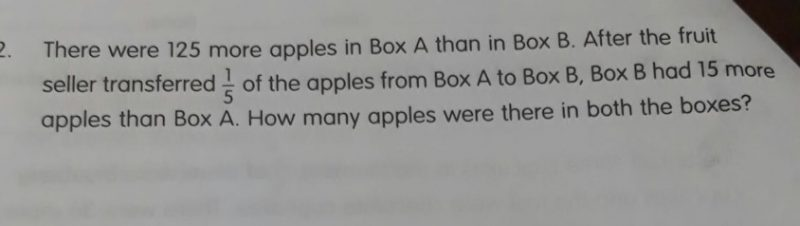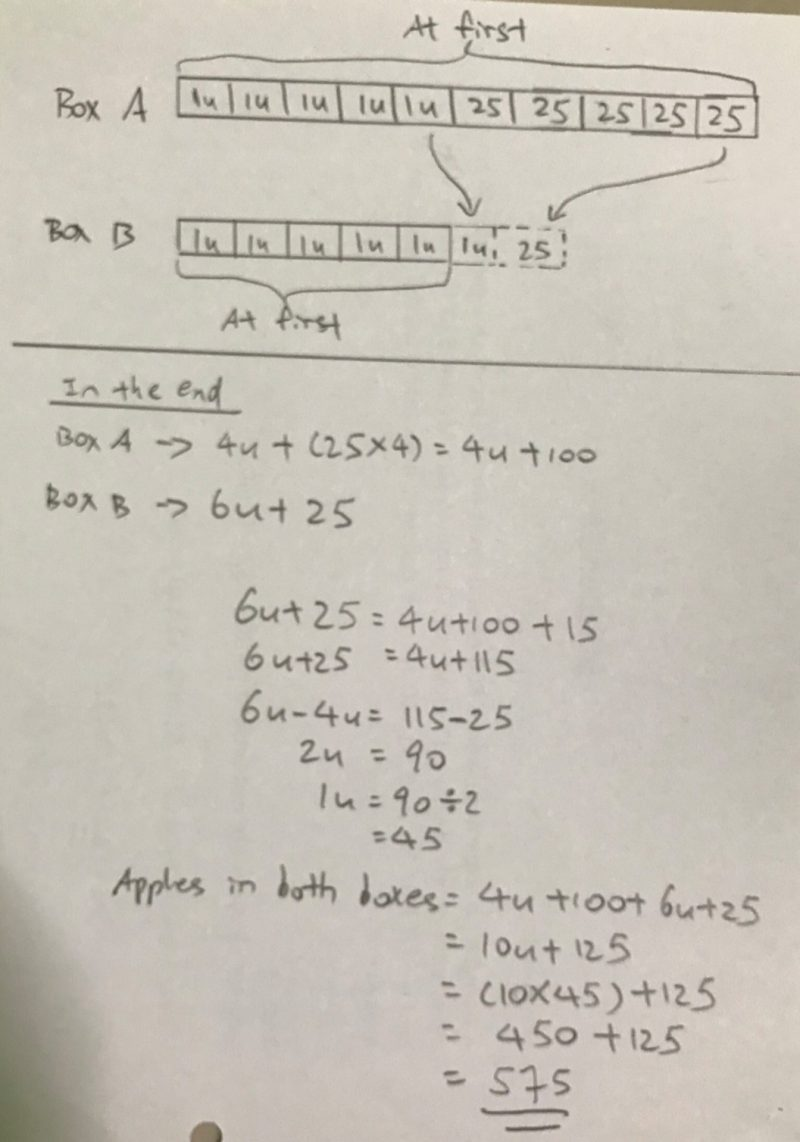# QuestionCan anyone please help to explain the above p5 problem sum ?

2 Answers

# Answer1 Reply 0 Likes

Thank you all for the solution . Really Appreciate your help

0 Replies 0 Likes

someone had asked this question, see my answer below:

assume box A has 5 unit,  at beginning, A = 5 unit, B = 5 unit -125,

after give 1/5, which is one unit,  A = 4 unit, B = 6 unit -125, and now B has 15 more apples, so 2 unit = 125+15, one unit is 70, box A has 350 apples

try to draw a simple model by this way, you should be able to get the answer

0 Replies 2 Likes